Get instant live expert help with Excel or Google Sheets“My Excelchat expert helped me in less than 20 minutes, saving me what would have been 5 hours of work!”

#### Post your problem and you’ll get expert help in seconds.

Your message must be at least 40 characters
Our professional experts are available now. Your privacy is guaranteed.

# How to Use the IFERROR Function in Excel

When you apply a formula in Excel it may return an error. Excel formulas return various error types due to a variety of reasons. You can use the IFERROR function to manage what happens when a mistake occurs.

### Possible Error Messages in Excel

The possible error messages from a formula could be due to;

• If expected value is not found as result of formula (#NA)
• Wrong data type present in cell used in formula (#VALUE!)
• When you divide a number by zero or an empty cell (#DIV/0!)
• When you use invalid cell references in formula (#REF!)
• When an incorrect range operator is used in formula (#NULL!)
• When formula contains invalid numeric values (#NUM!)

When you don’t want to show these returning errors as result of a formula in your data then Excel has a way to return an alternate value or custom value or blank cell. For this purpose IFERROR function is used to manage such errors.

### The IFERROR Function

By definition, Excel IFERROR function returns an alternate value if formula returns an error. It is categorized as a Logical function and it handles all types of errors including #N/A, #VALUE, #DIV/0, #REF!, #NUM!, #NULL or #NAME?.

The syntax for IFERROR function in Excel is as follows;

`IFERROR(value, value_if_error)`

Where,

• Value argument is what to check. It can be a formula, calculation, an expression, a value, or cell reference.
• Value_If_error argument is what to return. It contains the alternate value to return when the value argument returns an error. It can be an alternate formula, calculation, a blank cell, a numeric, or text value

When you apply the formula in this syntax, Excel checks and runs the value argument first and returns the resulting value of formula or calculation. If value argument returns an error then Excel returns or performs calculation supplied in value_if_error argument.

In this article, you will learn how to use IFERROR function in some basic and advance formula examples. This function is widely used to check and manage errors returned in functions and formulas like VLOOKUP, INDEX, and MATCH in Excel. It can be used to manage errors, ranging from basic calculations to array formulas and nested formulas to perform sequential value lookups. It is very handy to use and easy to manage.

### IFERROR in Basic Calculations

IFERROR function is very helpful to counter problems in basic calculations like division, multiplication, subtraction, and others. Suppose you have data for items’ cost and quantity and you want to calculate the price per unit by dividing cost price by quantity, like;

`=COST / QUANTITY`

When you apply this formula on data it may result in errors due to zero, blank or wrong data type values present in data cells. In order to manage those errors and return zero or blank cells where formula returns any type of errors, you need to use IFERROR function as shown below;

General formula result to calculate `Price/Unit = B3/C3`

IFERROR formula to calculate `Price/Unit= IFERROR(B3/C3,0)`

OR

IFERROR formula to calculate `Price/Unit= IFERROR(B3/C3,"")`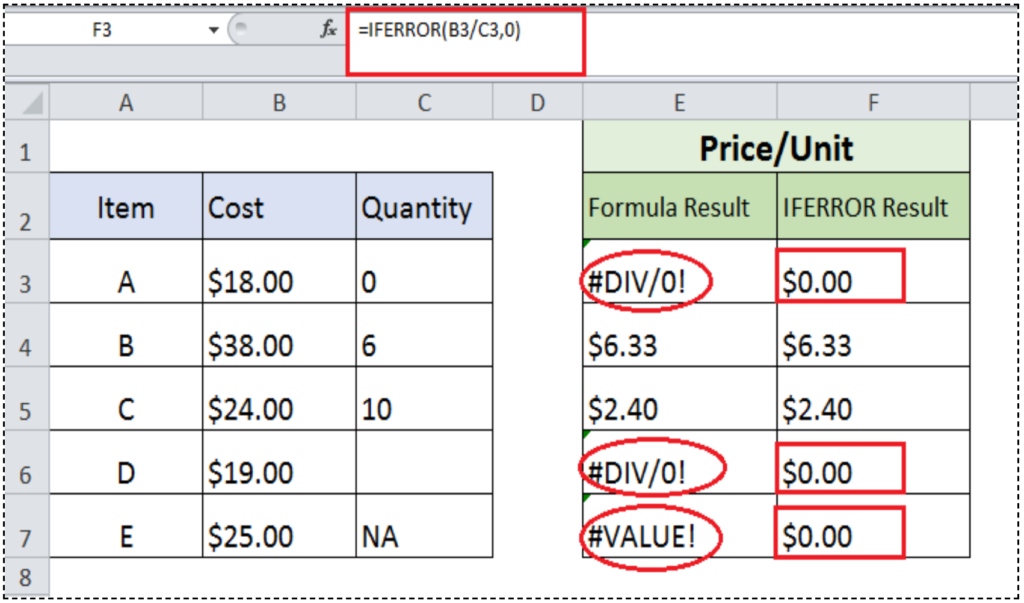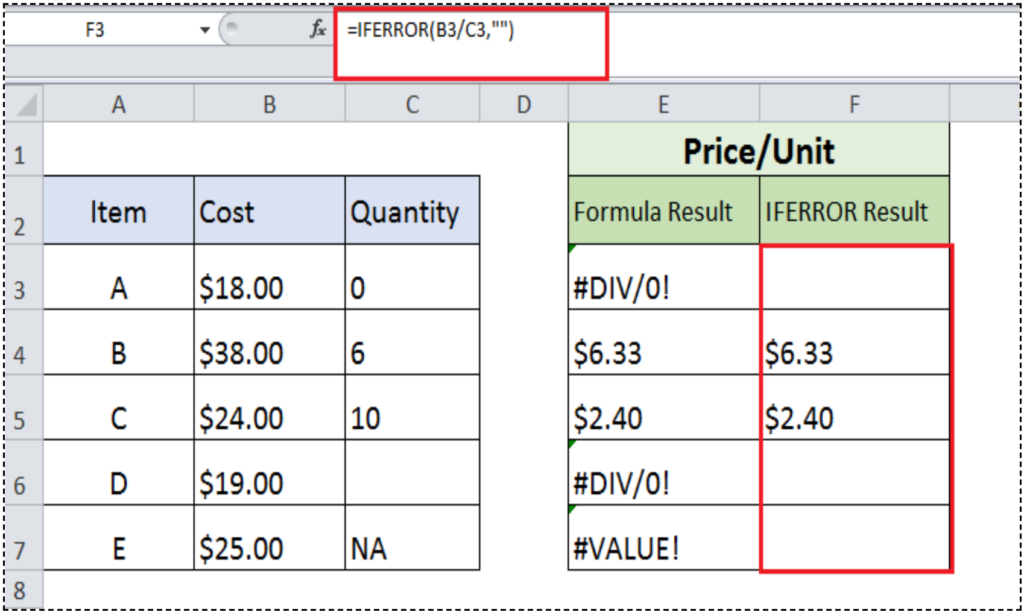### IFERROR with VLOOKUP Function

When you use VLOOKUP function to lookup a value in a table, generally it may return errors like #NA or #VALUE!, if VLOOKUP does not find required match value or value is of a wrong data type. In order to return an alternate value instead of an error value you need to use IFERROR function with VLOOKUP function. This alternate value could be another VLOOKUP function, a number or text value, a blank value or a custom text message alert. Syntax of such formula would be;

`IFERROR(VLOOKUP(),value_if_error)`

For example, you want to look up for amounts of various order numbers (Order_ID) from a table range G1:H6. When you use VLOOKUP function for this purpose then it returns #NA error where a value is not found, see in column C below. Whereas, if you use IFERROR function with VLOOKUP function to return a test string “Not Found” instead of #NA error then the formula would be;

`=IFERROR(VLOOKUP(A2,\$G\$1:\$H\$6,2,0),"Not Found")`

Copy down this formula in column D to other rows.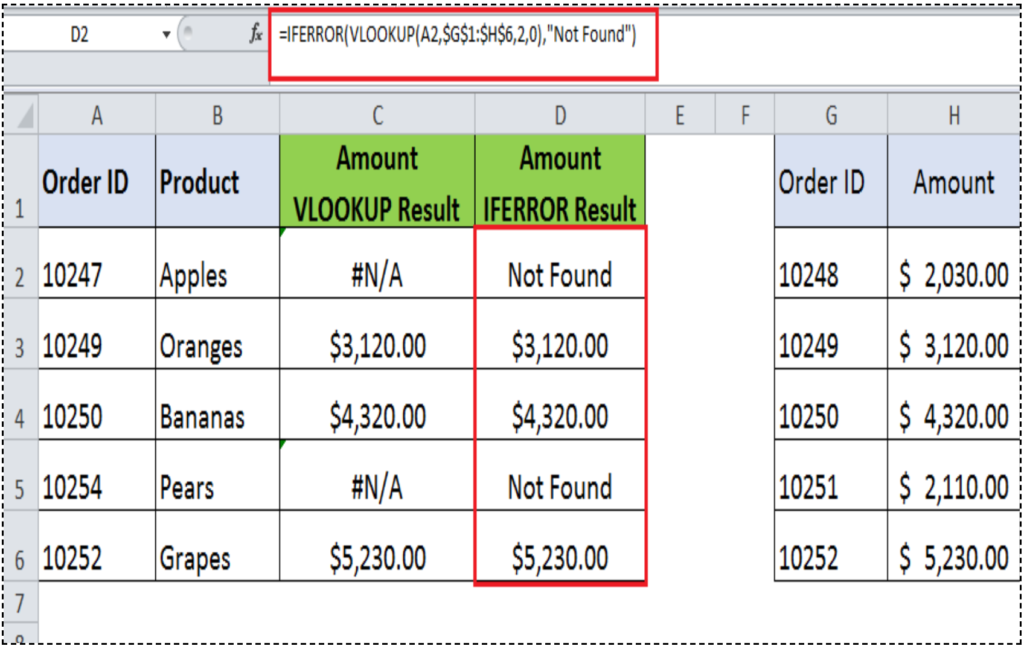### IFERROR with INDEX and MATCH Functions

If you want to look up for a value from a table using INDEX and MATCH function, it may return an error value if a value is not found in a table, same as in VLOOKUP case above. You can handle error value by using IFERROR function with INDEX and MATCH function together.

For the above example, the formula of IFERROR with INDEX and MATCH functions would be;

`=IFERROR(INDEX(\$H\$1:\$H\$6,MATCH(A2,\$G\$1:\$G\$6,0)),"Not Found")`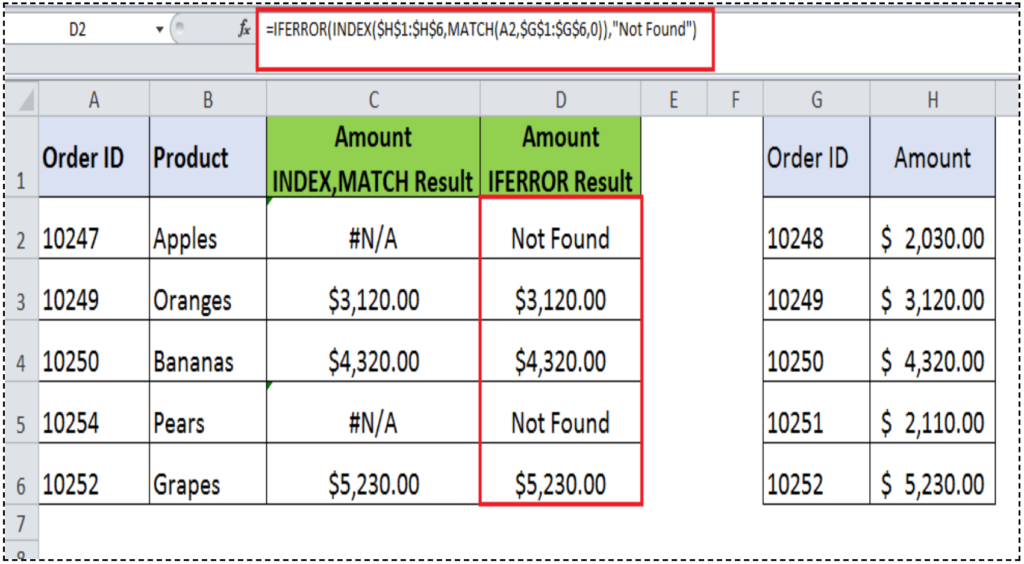### IFERROR function for VLOOKUPs on multiple tables

If you need to perform a value lookup from multiple tables at the same time then IFERROR function is the ideal function to perform this activity. You need to combine two or more IFERROR functions together to lookup for a value from two or more tables at the same time. In last IFERROR function, you need to supply alternate value to handle error message in the value_if_error argument if there is no match found.

The syntax of nested IFERROR functions for sequential Vlookups would be;

`IFERROR(VLOOKUP_1(),IFERROR(VLOOKUP_2(),......,value_if_error))`

For example, you have two separate tables containing price list of Fruits (E1:F12) and Vegetables (H1:I12) as shown below, and you need to sequentially lookup for a value in both tables to return its price if that value is found in any of two tables. And if a value is not found in any of both tables, then return value “Not Found”.

The formula for sequential VLOOKUPs would be;

`=IFERROR(VLOOKUP(A2,\$E\$1:\$F\$12,2,0),IFERROR(VLOOKUP(A2,\$H\$1:\$I\$12,2,0),"Not Found"))`

Still need some help with Excel formatting or have other questions about Excel? Connect with a live Excel expert here for some 1 on 1 help. Your first session is always free.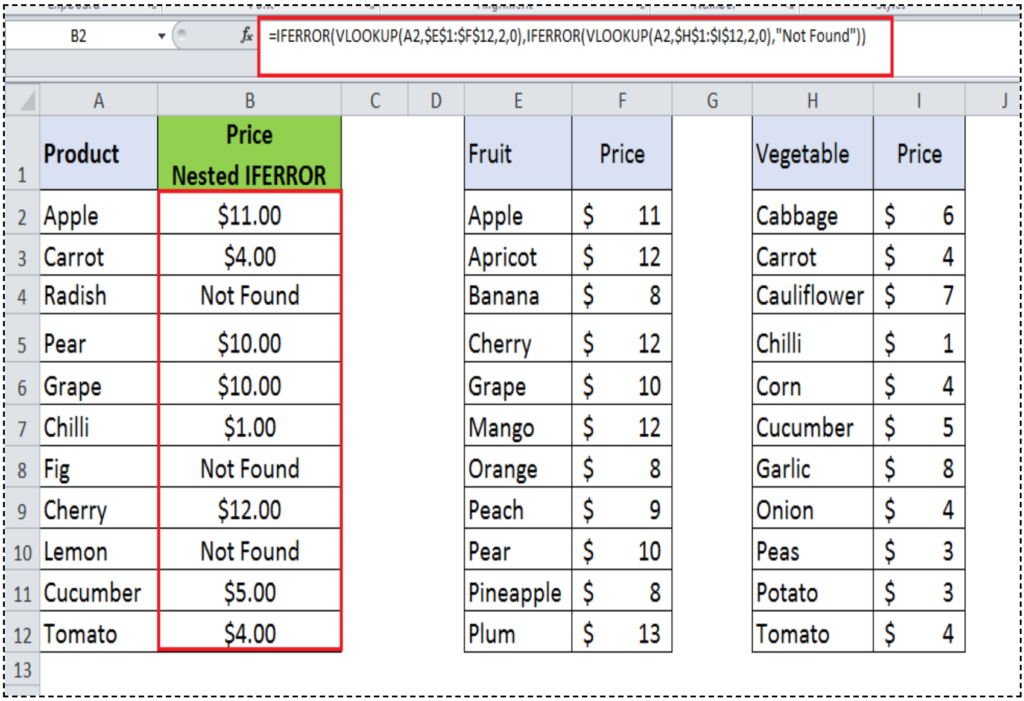Are you still looking for help with the IF function? View our comprehensive round-up of IF function tutorials here.

### Did this post not answer your question? Get a solution from connecting with the expert.Another blog reader asked this question today on Excelchat:
Solution examplesI need a formula that will compare multiple columns, and return the column header name of whichever column had the highest value. I am using (INDEX(\$F\$1:\$K\$1,0,MATCH(MAX(F2:K2),F2:K2,0))) , but one row had all the same values and the formula returned the first column header as being the "winner". This formula cannot distinguish when there are ties.
Solved by M. C. in 51 minsMy VLOOKUP formula won't work when value the "lookup cell" is derived from a formula. If I manually transfer the formula derived value to a different cell, then VLOOKUP formula works fine. What's going on?
Solved by Z. J. in 40 minsI'm having trouble with an index & match formula where I'm looking up two different pieces of info in order to return a specific value.
Solved by K. D. in 54 minsI have two lists. One is a list of part numbers. The other is a list of long text descriptions. I can use VLOOKUP with "*"&cell address"*" to find the first match. But how can I find the second and third matches? I have tried using INDEX and SHORT but the wild cards no longer find the match like they do in VLOOKUP. Can you help?
Solved by S. J. in 36 minsI have two columns with names and a third one with email addresses. For every name that matches, I need to copy the email address to an empty column next to the matching name. .
Solved by E. U. in 15 mins## Subscribe to Excelchat.coAnother blog reader asked this question today on Excelchat: